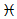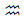2 . Indicator Parameters Synthesis Options:

Building Simple Waves and Trend Waves is not enough to open a position, that is why filtering potential market entry signals is introduced.

CompareWaveBLengths if true - in every Trend a Rollback wave length (4th, 6th, 8th, etc.) is compared to the previous Trend Wave Length in bars. The indicator calculates the ratio of each pair of waves Trend - Rollback . If the ratio of the current trend-rollback wave pair is greater than the previous one, opening orders is allowed

Green circles indicate the beginning of a rollback wave, after which the entrance is allowed. After the first rollback entry is always permitted.

The trend-rollback ratio of the second pair (red Fibo grid ) is smaller than the first pair (green Fibo grid ), therefore no entry is allowed.

The trend-rollback ratio of the third pair (blue Fibo grid ) is larger than the second pair (green Fibo grid ), therefore the input is allowed.

CompareWaveBHeights if true - the principle is the same as CompareWaveBLengths, but the Wave Heights are compared in pips.

The ratio Trend - Rollback of the second pair (red Fibo grid ) is larger than the first pair (green Fibo grid ), therefore no entry is allowed.

The Trend - Rollback ratio of the third pair Fibo blue grid ) is smaller than the second pair ( Fibo green grid ), so input is allowed.

ImpulsivityDeviation - if the value is greater than zero compares the “ Impulse ”coefficient of each wave. The formula for calculating the Wave Impulse Coefficient :

1.     Calculated Wave height in pips. Wave height waveHeight = High [MaxBar] - Low [MinBar]. MaxBar is a bar whose High price is the highest in the wave . MinBar - a bar whose Low price is the lowest in the wave .

2.     The height of each bar in the wave is calculated: barHeight = High-Low of each bar. The heights of all bars are summed up - barHeightSum .

3.      Wave Impulse Ratio ratio = waveHight / barsHeightSum .

Below are the calculations of the Impulse Coefficient ( ratio ) of each wave of the graph from left to right, the result of each wave is shown on the last bar of the corresponding wave:

Definition of the Impulse Structure and Rollback Structure: A Wave has a Impulse Structure if the condition is met:

Previous Wave Impulse Coefficient < ImpulsivityDeviation * Current Wave Impulse Coefficient.

If the wave does not have an impulse structure, then it has a rollback structure.

The indicator denotes a wave with an impulse structure symbol, with a rollback structure symbol.-        above the last bar of the wave, if the wave moves up

-        under the last bar of the wave, if the wave moves down

The picture shows an example with the parameter ImpulsivityDeviation = 1,

-          Ratio of the second wave (0.43) is less than the first (0.68) , the input is allowed.

-          Ratio of the fourth wave (0.59) is greater than the third (0.26) , entry is prohibited.

-          Ratio of the sixth wave (0.19) is less than the fifth (0. 71 ) , the input is allowed.

The goal of these filters is to avoid entering when the trend slows down, i.e. when the movement loses “strength”, the movement towards the Trend is longer in time and shorter in the number of pips.

To be continued.

The indicator is published on the market https://www.mql5.com/en/market/product/33478

Share it with friends: